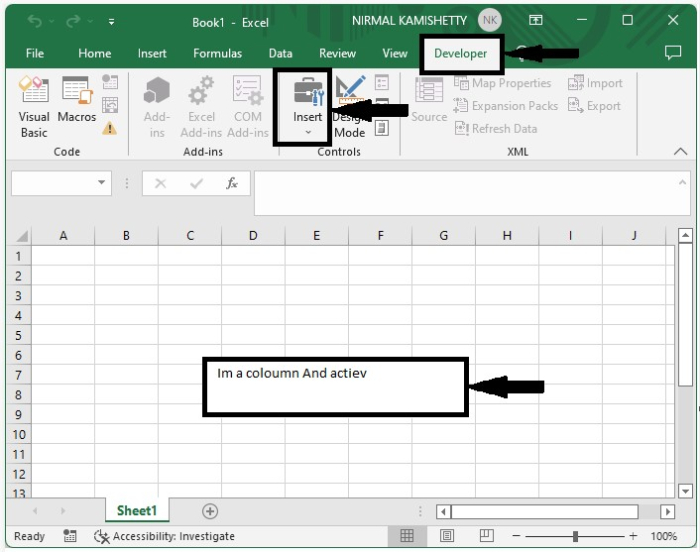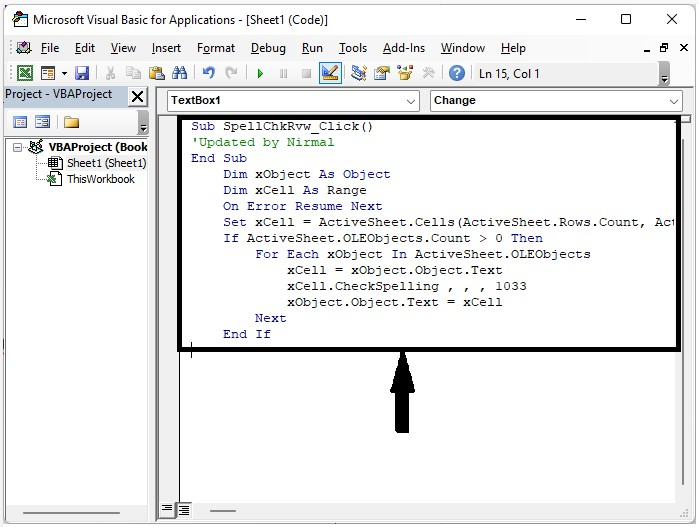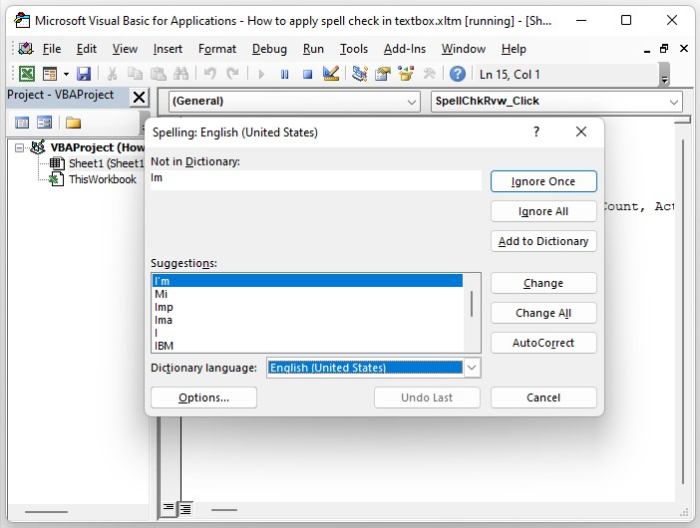# How to Apply Spell Check in Excel Textbox?

We frequently use the "spell check" feature in Word, but have you ever tried to apply spell check for textboxes in Excel? This is not an inbuilt process for Excel; hence, we need to modify the textbox in order to complete our process. The spell check can’t be applied to normal textboxes; it needs to be an ActiveX command textbox, which will be present under the developer option in Excel.

Read this tutorial to understand how you can apply spell check in an Excel textbox.

## Applying Spell Check in Excel Textbox

Here we will first insert a VBA module and then run it to complete the task. Let us see a simple process to understand how we can apply spell check for textboxes in Excel. We will be using the help of the VBA application in order to complete our process.

Step 1

Let us consider an Excel sheet where an ActiveX command textbox is present, as shown in the below image. To enter the data into the textbox, exit the design mode and then enter the data.To use the spell check, exit design mode, then right-click on the textbox and select view code to open the VBA application, then replace the existing code with the programme shown in the figure below.

### Program

Sub SpellChkRvw_Click()
'updated by Nirmal
Dim xObject As Object
Dim xCell As Range
On Error Resume Next
Set xCell = ActiveSheet.Cells(ActiveSheet.Rows.Count, ActiveSheet.Columns.Count)
If ActiveSheet.OLEObjects.Count > 0 Then
For Each xObject In ActiveSheet.OLEObjects
xCell = xObject.Object.Text
xCell.CheckSpelling , , , 1033
xObject.Object.Text = xCell
Next
End If
End SubStep 2

Now save the sheet with macros enabled, then click on F5 to run the code and complete our process as shown in the below image.## Conclusion

In this tutorial, we used a simple example to demonstrate how we can apply spell check to textboxes in Excel to highlight a particular set of data.